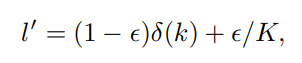# Label Smooth Regularization using KD_Lib¶

Paper

• Considering a sample x of class k with ground truth label distribution l = δ(k), where δ(·) is impulse signal, the LSR label is given as -where K is the number of classes

To use the label smooth regularization with incorrect teacher predictions replaced with labels where the correct classes have a probability of 0.9 -

import torch
import torch.nn as nn
import torch.optim as optim
from torchvision import datasets, transforms
from KD_Lib.KD import LabelSmoothReg

# Define datasets, dataloaders, models and optimizers

datasets.MNIST(
"mnist_data",
train=True,
transform=transforms.Compose(
[transforms.ToTensor(), transforms.Normalize((0.1307,), (0.3081,))]
),
),
batch_size=32,
shuffle=True,
)

datasets.MNIST(
"mnist_data",
train=False,
transform=transforms.Compose(
[transforms.ToTensor(), transforms.Normalize((0.1307,), (0.3081,))]
),
),
batch_size=32,
shuffle=True,
)

# Set device to be trained on

device = torch.device("cuda:0" if torch.cuda.is_available() else "cpu")

# Define student and teacher models

# Define optimizers

teacher_optimizer = optim.SGD(teacher_model.parameters(), lr=0.01)
student_optimizer = optim.SGD(student_model.parameters(), lr=0.01)

# Train using KD_Lib